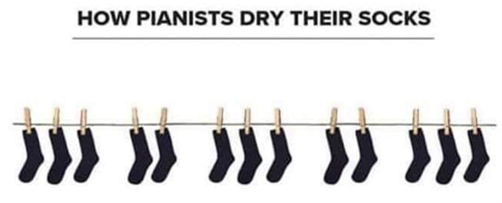# Problem of the Week## Peg Pattern

If there is 10cm between consecutive pegs within a group of 2 or 3 pegs, and there is 15cm between groups, how far is it between the first peg and the last peg pictured here? How did you work it out? If this pattern continues for 100 socks, what will be the distance between the first and last peg?

How far is it between the first peg and the last peg?

• Going from left to right: 10 + 10 + 15 + 10 + 15 + 10 + 10 + 15 + 10 + 15 + 10 + 10 = 140 cm
• This could also be seen as: 10 x 8 + 15 x 4 = 80 + 60 = 140 cm
• or it could be seen as (10 + 10 + 15 + 10 + 15) x 2 + 10 + 10 = 60 x 2 + 10 + 10 = 140 cm

If this pattern continues for 100 socks, what will be the distance between the first and last peg?

See what the distance is after each group of 5 socks:  10 + 10 + 15 + 10 + 15 = 60 cm

100 socks is 20 groups of 5 socks: 60 cm x 20 = 1200 cm

However the last group of 5 socks does not include a 15 cm space at the end,

so the distance between the first and last peg = 1200 – 15 cm = 1185 cm